# Test Prep Plan - Take a practice test

Take this practice test to check your existing knowledge of the course material. We'll review your answers and create a Test Prep Plan for you based on your results.
How Test Prep Plans work
1
2Based on your results, we'll create a customized Test Prep Plan just for you!
3Study smarter
Study more effectively: skip concepts you already know and focus on what you still need to learn.

# Derivative Rules Chapter Exam

Exam Instructions:

Choose your answers to the questions and click 'Next' to see the next set of questions. You can skip questions if you would like and come back to them later with the yellow "Go To First Skipped Question" button. When you have completed the practice exam, a green submit button will appear. Click it to see your results. Good luck!

### Page 1

#### Question 1 1. What is the value of f(x) at the red circle?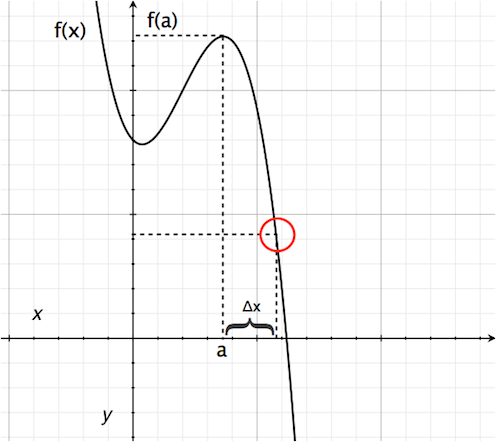#### Question 2 2. Which is greater, the derivative at a or the derivative at b?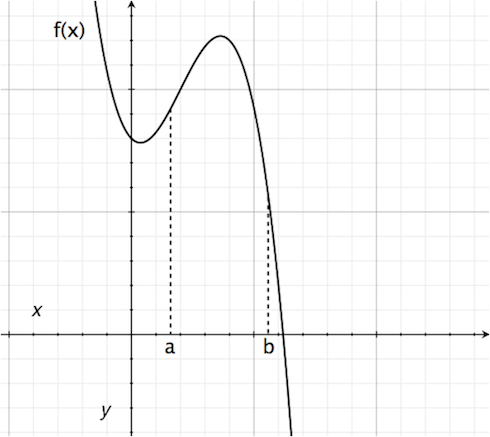#### Question 3 3. The derivative of f(x) is equal to 0 at how many points on the graph below?#### Question 4 4. Consider the graph and question below..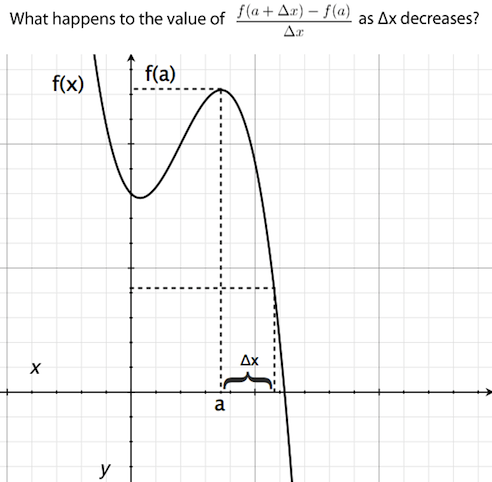#### Question 5 5. Find f'(t) given the function f(t):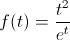### Page 2

#### Question 6 6. Differentiate the following function: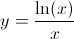#### Question 7 7. Evaluate the following: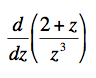#### Question 8 8. Find y'.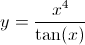#### Question 9 9. What is the derivative of f(x)?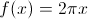#### Question 10 10. What is the derivative of g(t)? Assume C is a constant.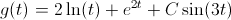### Page 3

#### Question 11 11. What is the derivative of f(z)?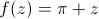#### Question 12 12. What is the derivative of f(x)?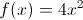#### Question 14 14. What is the derivative of the following?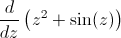#### Question 15 15. Where t= time, what is the derivative of f(t)?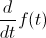### Page 4

#### Question 17 17. Calculate the derivative of f(x), and evaluate it at x=2.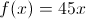#### Question 18 18. The derivative below may have been calculated from which function f(x)?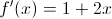#### Question 19 19. Which of the following statements is true about the derivative of f(x)?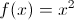#### Question 20 20. Calculate the derivative of g(t).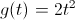### Page 5

#### Question 21 21. If h(t) is the height of an object as a function of time, then which of the following statements are true about h(t)? (Assume t>0.) A) When hitting the ground (at h(t)=0), the object's velocity is -2. B) When hitting the ground (at h(t)=0), the object's acceleration is -2. C) The object hits the ground at t=2.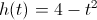#### Question 22 22. The graph x(t) represents the position of an object as a function of time. What is the acceleration of the object for t>0?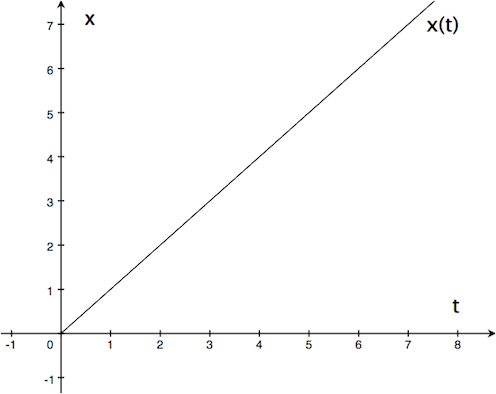#### Question 23 23. The function v(t) represents the velocity of an object as a function of time. Given the graph of v(t) below, which of the following statements is true?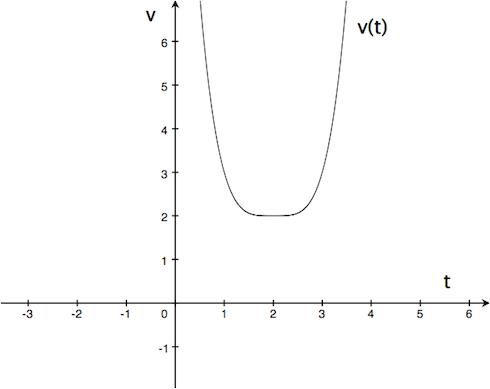#### Question 24 24. If the following graph is f(x), then which of the graphs below could be f''(x)?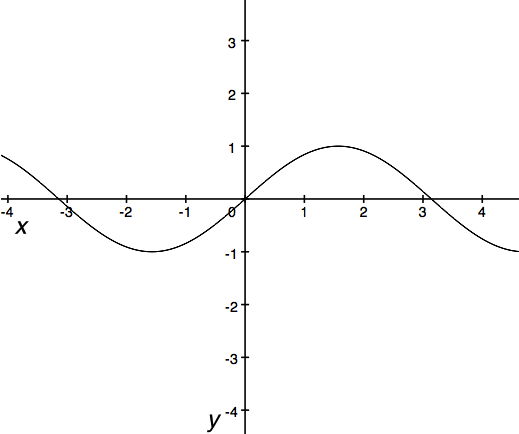### Page 6

#### Question 26 26. The derivative of the following graph is _____ at point C.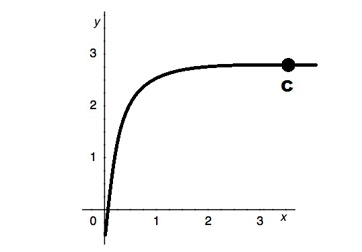#### Question 27 27. The derivative of the following graph is _____ at point D.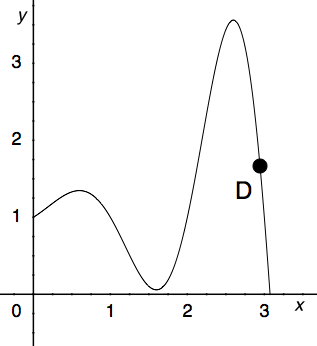#### Question 28 28. Which could be the graph of the derivative of the function below?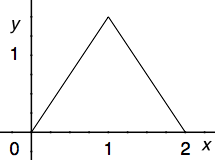#### Question 29 29. At how many points on the graph below is the derivative of f(x)=0?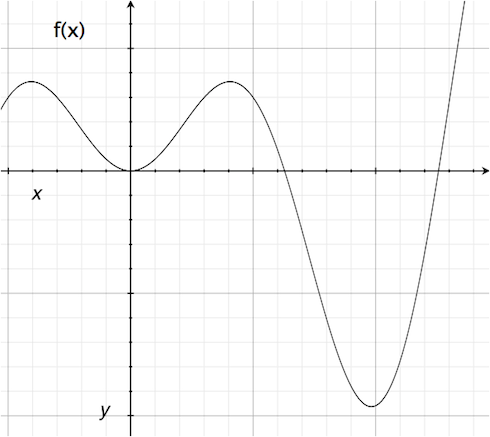#### Question 30 30. Find the derivative of f(x).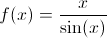#### Derivative Rules Chapter Exam Instructions

Choose your answers to the questions and click 'Next' to see the next set of questions. You can skip questions if you would like and come back to them later with the yellow "Go To First Skipped Question" button. When you have completed the practice exam, a green submit button will appear. Click it to see your results. Good luck!

Support